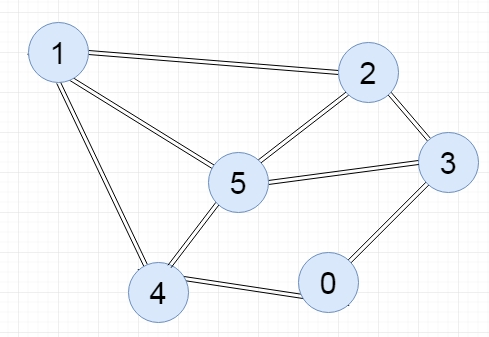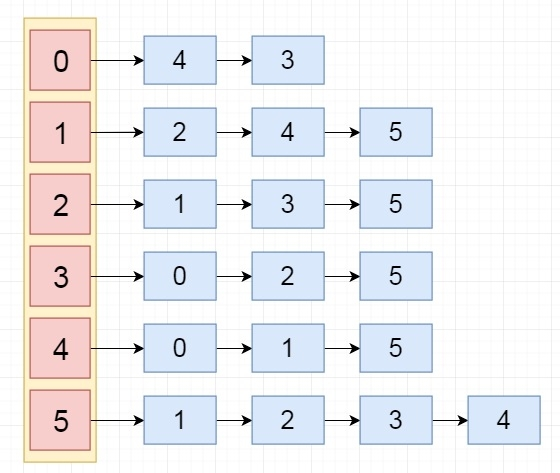# C++ Program to Represent Graph Using Linked List

The incidence matrix of a graph is another representation of a graph to store into the memory. This matrix is not a square matrix. The order of the incidence matrix is V x E. Where V is the number of vertices and E is the number of edges in the graph.

In each row of this matrix we are placing the vertices, and in each column the edges are placed. In this representation for an edge e {u, v}, it will be marked by 1 for the place u and v of column e.

## The complexity of Adjacency Matrix representation

• The incidence matrix representation takes O(V x E) amount of space while it is computed. For complete graph the number of edges will be V(V-1)/2. So incidence matrix takes larger space in memory.

Input:Output:## Algorithm

Input − The u and v of an edge {u,v}, and the adjacency list

Output − Adjacency List of the graph G

Begin
Append v into the list at index u
Append u into the list at index v
End

## Example Code

Live Demo

#include<iostream>
#include<list>
#include<iterator>
using namespace std;
for(int i = 0; i<v; i++) {
cout << i << "--->";
list<int> :: iterator it;
cout << *it << " ";
}
cout << endl;
}
}
void add_edge(list<int> adj_list[], int u, int v) {    //add v into the list u, and u into list v
}
main(int argc, char* argv[]) {
int v = 6; //there are 6 vertices in the graph
//create an array of lists whose size is 6
}
0--->4 3
5--->1 2 3 4